Trial ends in

A subscription to JoVE is required to view this content.
You will only be able to see the first 20 seconds.

Conservation of Momentum
##### Science Education (Physics I)
Force and Acceleration
Torque

# Conservation of Momentum

### Overview

Source: Nicholas Timmons, Asantha Cooray, PhD, Department of Physics & Astronomy, School of Physical Sciences, University of California, Irvine, CA

The goal of this experiment is to test the concept of the conservation of momentum. By setting up a surface with very little friction, collisions between moving objects can be studied, including their initial and final momenta.

The conservation of momentum is one of the most important laws in physics. When something is conserved in physics, the initial value is equal to the final value. For momentum, this means that the total initial momentum of a system will be equal to the total final momentum. Newton's second law states that the force on an object will be equal to the change in the object's momentum with time. This fact, combined with the idea that momentum is conserved, underpins the workings of classical mechanics and is a powerful problem-solving tool.

### Principles

Momentum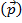is defined as the mass of an object times its velocity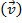: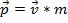. (Equation 1)

One can also define momentum in terms of the forces acting upon an object (Newton's second law):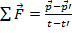. (Equation 2)

Here,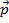is the initial momentum andis the final momentum, with the same convention used for timeand. The sum of the forces acting upon an object is equal to the change in the object's momentum with time. Therefore, if there is no net force acting upon an object, the change in the momentum will be zero. Said another way, in a closed system with no external forces, the initial momentum will be equal to the final momentum.

This concept is most easily understood in the context of one-dimensional and two-dimensional collisions. In one-dimensional collisions, an object with mass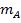and initial velocitycollides with another object with mass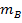and initial velocity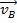. In these collisions, external forces will be assumed to be too small to have an effect. In the lab, an air track is used to reduce the amount of friction, an external force, on the gliders. If the initial momentum is equal to the final momentum, then: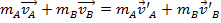, (Equation 3)

where the primed velocities represent that final velocities and the unprimed velocities represent the initial velocities.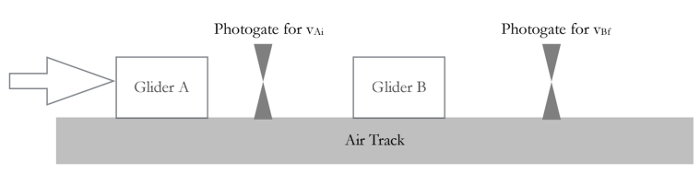Figure 1. Experimental Setup.

### Procedure

1. Understanding the photogate timer.

1. Using a balance, measure and record the mass of each glider.
2. Place one glider on the track with one photogate timer.
3. Set the photogate timer to the "gate" setting.
4. When the glider passes through the photogate, it will record the time at which the flag above the glider passes through the gate. On a return trip, the photogate will not display a new time. Switch the toggle to "read" so that it will display the initial time plus the time of the second pass through the gate.
5. The flag is 10 cm long; determine the velocity of the glider using the fact that the speed is distance divided by time.
6. Send the glider through the photogate several times, including the return trips after it has bounced off the far wall, and measure the velocities in order to become familiar with the equipment. Remember that velocity has a direction. Let the initial velocity direction represent positive and the opposite direction represent negative velocity values.

2. Two gliders of equal mass.

1. Place two gliders and two photogate timers on the track, as in Figure 1.
2. Use Equation 3 to determine the expression for the final velocities. In this part of the experiment, glider B will start from rest.
3. Give glider A some initial velocity so that it will collide with glider B. Record the initial velocity of glider A, as well as the final velocities of each glider. Do this three times, record your results, and compare them to the theoretical prediction.

3. Two gliders of unequal mass.

1. Add 4 weights to glider B, which will double its mass. Repeat steps 2.1-2.3.

4. Equal masses not starting from rest

1. Remove the weights from glider B.
2. Repeat steps 2.1-2.3, but give glider B an initial velocity as well, in the direction of glider A.

Conservation of momentum is one of the most important laws in physics and underpins many phenomena in classical mechanics.

Momentum, typically denoted by the letter p, is the product of mass m and velocity v. The principle of momentum conservation states that an object's change in momentum, or Δp, is zero provided no net external force is applied.

Conversely, applying a net external force, or F net, over a period of time results in a change in momentum for that object. The phenomenon of momentum conservation can also be applied to a collection of objects, which makes it useful for studying the physics of collisions.

The goal of this experiment is to test the principle of conservation of momentum by observing collisions between moving objects.

Before delving into the lab experiment, let's study the basic principles of momentum conservation. Newton's laws of motion are central to understanding the principle of momentum conservation. For more information, please watch JoVE's Science Education video: Newton's Laws of Motion.

The concepts of momentum can be illustrated using a cue ball on a pool table. Newton's second law states that a net force applied by a cue stick imparts an acceleration a to a cue ball of mass m. Acceleration is the change in velocity v over time t. So, if we move time to the other side of the equation, we are left with Δmv, or the change in momentum Δp. Therefore, the net force gives rise to a change in momentum.

Note that the m in this equation is typically constant, so the change in momentum is dependent on the difference in velocities at the final and initial reference points. And since velocity is a vector quantity, a positive or negative sign is attributed to its value which indicates direction of motion.

In the cue ball example, the initial velocity at point A -- denoted by vA in this equation -- is zero. Whereas the final velocity at point B is positive. Thus, the momentum change is positive due to the net force applied by the stick. Then, when the ball is moving from point B to point C, assuming that there are no external forces acting on the ball like friction or air resistance, Δp would be zero.

Note that momentum can only be conserved in an isolated system - a system unaffected by net external forces.

Now, when the cue ball moves from point C and strikes the side of the table at point D, its final velocity becomes zero. Thus, the momentum change becomes negative while retaining the same magnitude as when the ball was struck by the cue stick. Lastly, when the cue ball rebounds off the wall, its final velocity at point E is negative due to change in direction. We know that the initial velocity at point D is zero, therefore the change in momentum remains negative because of the change in direction of movement.

This phenomenon of momentum change and conservation is useful for studying collisions as well, like between two pool balls. Note that in this case the two balls together would be treated as an isolated system. Therefore, the sum of the bodies' initial momenta before the collision would equal the sum of their final momenta afterwards. Also, the momentum change of one body would be equal and opposite to that of the other - reflecting Newton's third law.

Note that these pool ball collisions would be considered elastic, meaning that both momentum and kinetic energy or KE, of the system, are conserved; but this is not the case always. In fact, more commonly encountered collisions, such as car crashes, are inelastic and may not obey momentum conservation because some kinetic energy is lost during impact.

Now that we have reviewed the principles of momentum conservation, let's see how these concepts can be applied to an experiment involving collisions of gliders on a near frictionless track.

This experiment consists of a balance, two photogate timers, two gliders of equal mass, additional weights, an air supply, an air track with bumpers, and a ruler.

First, using the balance, measure the masses of the gliders, the additional weights, and record these values. Next, connect the air supply to the air track and turn it on. An air track is used to reduce the amount of friction, which would be an external force on the gliders.

Now begin familiarizing yourself with the timing process by placing one glider and a component of one of the photogate timers on the track. Set the timer to the 'gate' setting and push the glider toward the photogate. When the flag above the glider passes through the photogate it will record its transit time. Knowing the flag is 10 centimeters long, divide this distance by the measured time to get the velocity of the glider.

The glider will bounce off the far bumper and return to pass through the photogate again. The photogate displays the initial transit time and can be switched to the 'read' setting to display the return transit time. Repeat the process of measuring the velocity of the glider during the initial and return trips to familiarize yourself with the process. Since velocity is a vector quantity, let the initial direction be positive and the return direction be negative.

Place a second glider and photogate timer on the track to the right of the first set. With glider 2 at rest, push glider 1 so that the two will collide. Record the initial velocity of glider 1 as well as the final velocities of each glider. Note that the momenta are being measured after the impulsive force has been applied and the system is isolated. Repeat this procedure three times to get multiple data sets.

Next, with the gliders in their original positions, place an additional set of weights on glider 2 that doubles its mass. Repeat the previous set of velocity measurements for this mass configuration and record these values.

Lastly, reset the gliders to their original positions and remove the additional weights from glider 2. For this set of measurements, glider 2 will be given an initial velocity such that both gliders will receive a push prior to the collision. Record the initial and final velocities for each glider and repeat this procedure three times.

For the first experiment involving equal masses and glider 1 initially moving, glider 1 comes to almost a complete stop after colliding with glider 2. And the velocity of glider 2 after collision is similar to the velocity of glider 1 before collision. Thus, the change in momentum of one glider is equal and opposite to the momentum change of the other, which makes this a good example of Newton's 3rd Law

As expected, the initial and final momenta of the whole system are nearly equal, reflecting conservation of momentum. Discrepancies in these momenta values are consistent with errors expected for this type of experiment including measurement error and the track not being completely level.

For the second experiment involving unequal masses, glider 1 does not come to rest following the collision with the heavier glider, but reverses direction after imparting some momentum to glider 2.

Once again, the momentum changes of the gliders are equal and opposite while the momentum of the total system is conserved. The system momentum as well as its initial and final kinetic energies are nearly conserved. This is because the collision is nearly elastic and therefore negligible external friction forces are present.

For the third experiment involving gliders of equal mass moving in opposite directions, the gliders possess similar initial momenta and then reverse their directions after colliding while retaining their magnitudes of momenta.

The total system momentum is conserved although the discrepancies in the initial and final momentum values are slightly larger than the previous experiments owing to the additional velocity measurement required and potentially larger losses due to friction.

The principle of conservation of momentum, while not typically considered, is prominent in all manners of activities and events. Without momentum conservation rocket propulsion would not be possible. Initially the rocket and its fuel are motionless and have zero momentum.

However, by rapidly expelling spent fuel that has both mass and momentum, the rocket is propelled upward, as a result of the momentum in the opposite direction of the discarded fuel. This explains how rockets can create thrust and propel in air or space without pushing against anything.

The discharge of a firearm has a notable association with the conservation of momentum.

Like the rocket-fuel system, the firearm-ammunition system also starts at rest. When the ammunition is fired out of the firearm at a tremendous speed, there has to be opposing momentum to counter it. This is known as recoil and can be very powerful.

You've just watched JoVE's introduction to Conservation of Momentum. You should now understand the principle momentum conservation and how this can be applied to solve problems and understand the physics of collisions. As always, thanks for watching!

### Results

Table 1. Results from two gliders of equal mass.

 Glider (trial)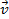(cm/s)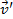(cm/s)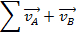(cm/s)(cm/s) Difference (%) A (1) 72.5 -0.2 - - - B (1) 0.0 67.1 72.5 66.9 8 A (2) 35.6 0.3 - - - B (2) 0.0 37.4 35.6 37.7 6 A (3) 47.4 0.0 - - - B (3) 0.0 47.8 47.4 47.8 1

Table 2. Results from two gliders of unequal mass.

 Glider (trial)(cm/s)(cm/s)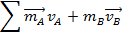(kg cm/s)(kg cm/s) Difference (%) A (1) 52.9 -10.7 - - - B (1) 0.0 37.7 52.9 64.7 22 A (2) 60.2 -13.2 - - - B (2) 0.0 41.5 60.2 69.8 16 A (3) 66.2 -12.0 - - - B (3) 0.0 45.9 66.2 79.7 20

Table 3. Results from equal masses not starting from rest.

 Glider (trial)(cm/s)(cm/s)(cm/s)(cm/s) Difference (%) A (1) 48.8 -29.9 - - - B (1) -42.4 39.8 6.4 9.9 55 A (2) 38.6 -25.2 - - - B (2) -33.4 32.8 5.2 7.6 46 A (3) 38.9 -43.1 - - - B (3) -48.5 36.3 -9.6 -6.8 41

The results for steps 2, 3, and 4 confirm the predictions made by Equation 3. In step 2, glider A comes to an almost complete stop after colliding with glider B. Therefore, nearly all its momentum is transferred to glider B. In step 3, glider A does not come to a stop after colliding with the heavier glider B. Instead, it returns in the opposite direction after imparting some momentum unto glider B. In step 4, the total momentum of the system remains the same, despite the direction changes of both gliders. The fact that, in some cases, the total momentum seems to increase and the velocities of both gliders decrease is related to the fact that there is experimental error and the collisions themselves are not completely elastic. Sound and heat given off by the collisions can take energy away from the system. The fact that the air track might not be totally level can change the behavior of the velocities of the gliders. If the track is even slightly inclined, the velocities will increase in that direction due to gravity. The results still show that the total momentum of the system, regardless of initial velocities, remains constant.

### Applications and Summary

Without momentum conservation, rockets would never leave the ground. Rockets do not actually push against anything-they rely on thrust to lift off. Initially, the fuel of a rocket and the rocket itself are motionless and have zero momentum. When launching, the rocket propels spent fuel out very rapidly. This spent fuel has mass and momentum. If the final momentum must be equal to the initial momentum (zero) then there must be some momentum in the opposite direction of the discarded fuel. Thus, the rocket is propelled upward.

Anyone who has ever fired a gun understands the conservation of momentum. Like the rocket/fuel system from above, the gun/ammunition system also starts at rest. When the ammunition is fired out of the gun at a tremendous speed, there must be some momentum in the opposite direction to cancel out the momentum of the speeding bullet. This is known as recoil and can be very powerful.

The popular desk ornament that consists of several metal balls hanging from strings is called a "Newton's cradle" for good reason. It is another example of the conservation of momentum. When a ball is lifted and released, it strikes its neighbor, transferring its momentum. The momentum travels down the line until the final ball has the momentum of the first, causing it to swing outward. This would go on forever if not for outside forces, such as air resistance and energy loss due to the collisions.

In this experiment, the law of conservation of momentum was verified by considering the collision of two gliders on a near-frictionless track. This fundamental law is perhaps most important because of its power to solve problems. If someone knows the initial momenta, then she knows the final momenta, and vice versa.X